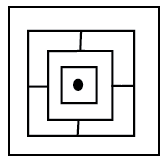Question 195

# Find out which of the answer figures will exactly make up the question figure ?Question Figure :Solution

In the question figure, there are two outer squares which are given in all the options, if we look inside it, we find :

two squares of different length, one black dot, and four straight lines of length less than the smaller square of the two.

Thus we need : 12 straight lines(4*2+4) in group of 3( each group of different lengths) and one black dot which is clearly given in option (A)

Ans - (A)

• Free SSC Study Material - 18000 Questions
• 230+ SSC previous papers with solutions PDF